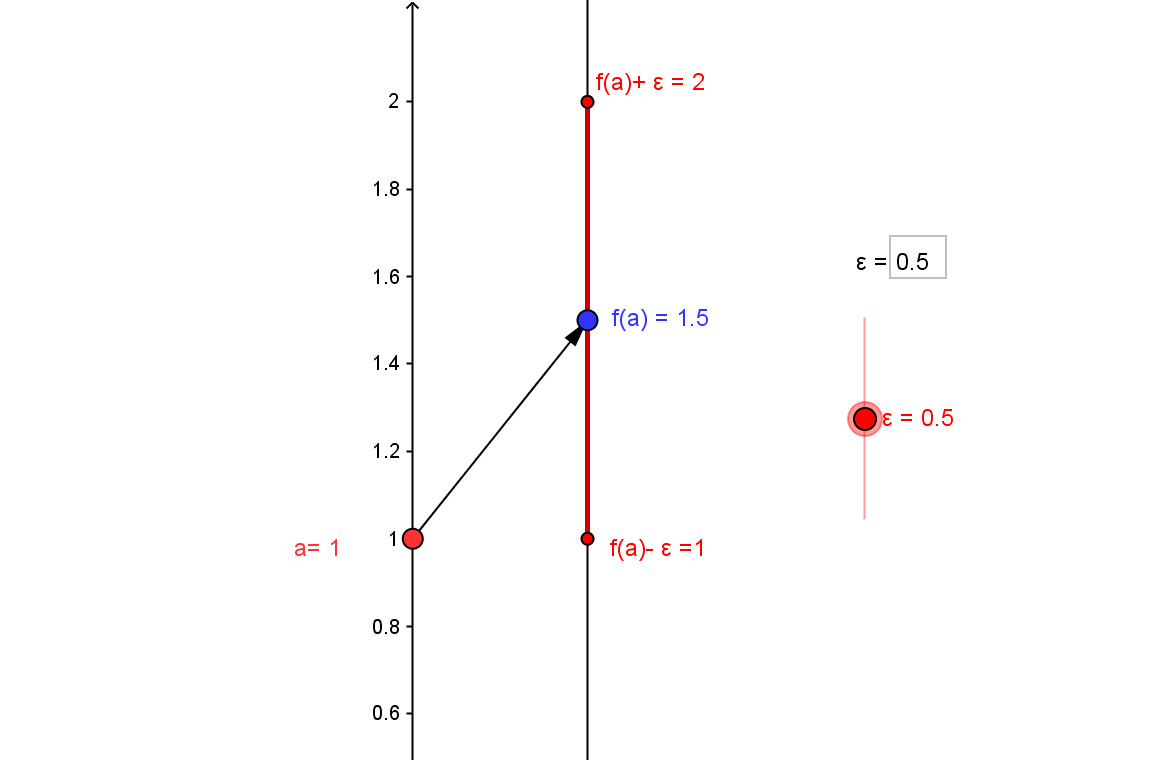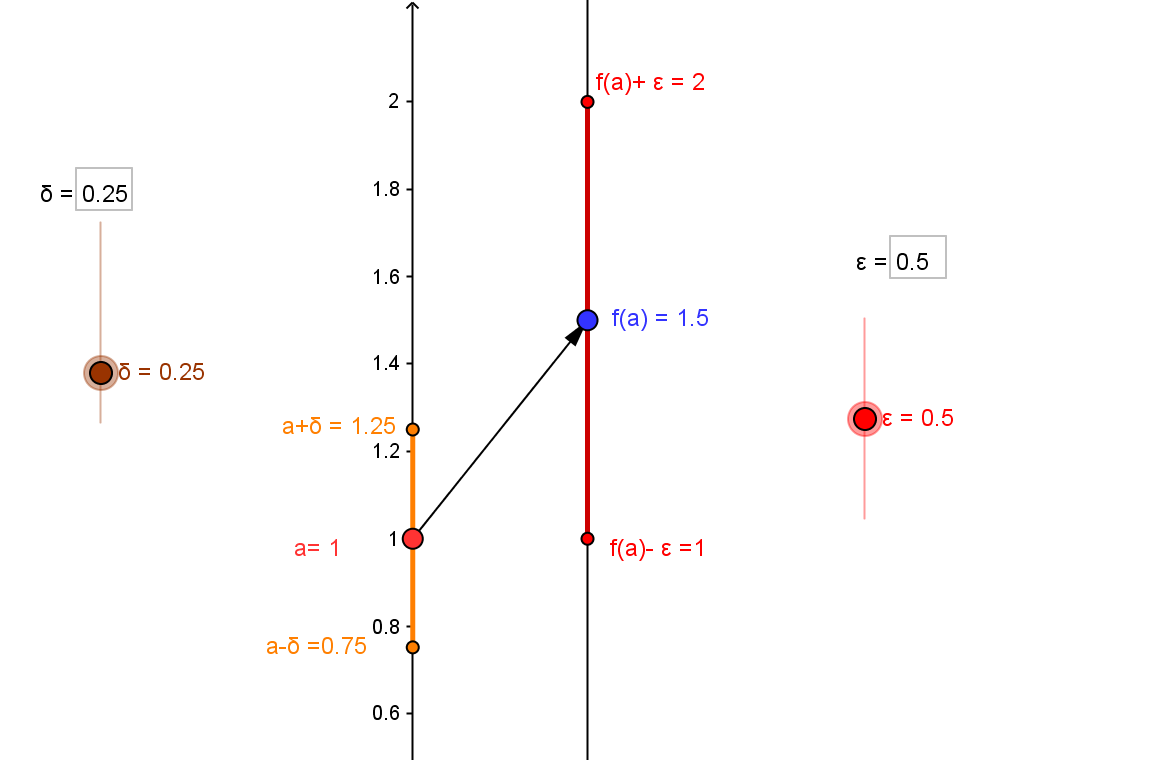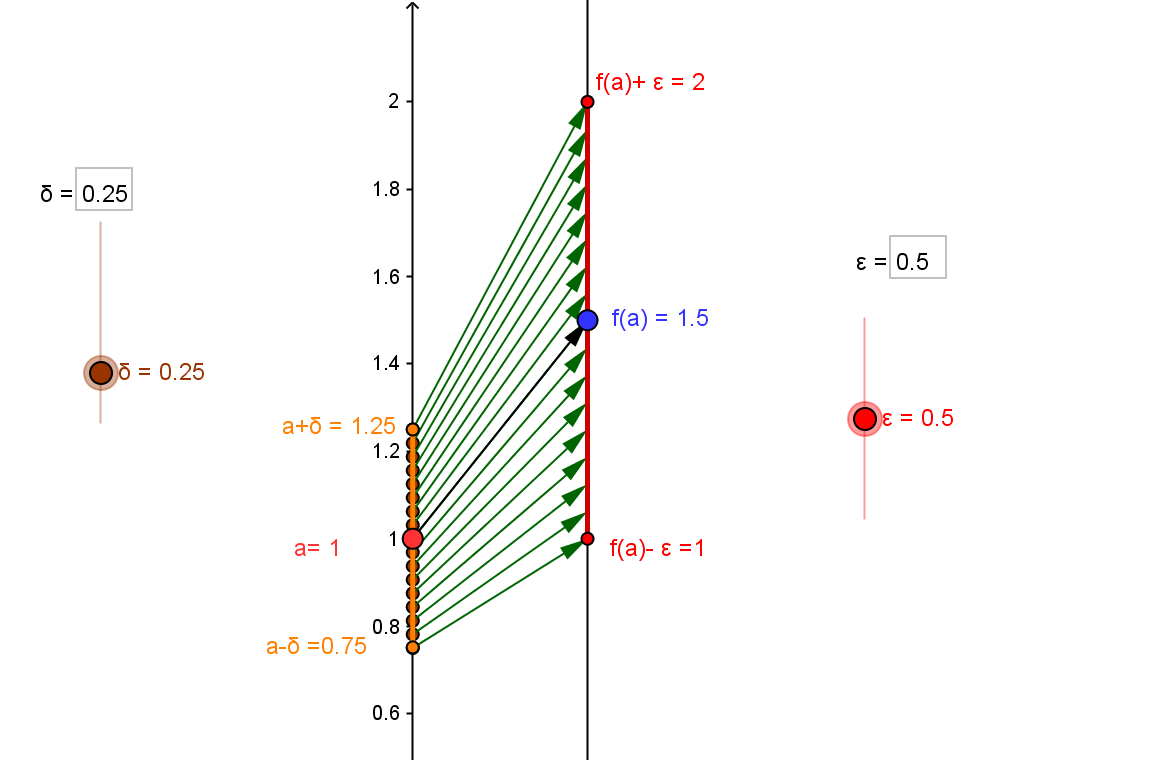Definition of Continuity (at a Point): CCD.DCP
A function $f$ is defined to be continuous at the number $a$ provided that $\lim_{x \to a} f(x) = f(a)$, i.e., $f$ is defined for all $x$ in an open interval containing $a$ and the values $f(x)$ approach $f(a)$, ($f(x) \to f(a)$).
The continuity of $f$ at $a$ is interpreted traditionally as...
1. The value $f(a)$ is the best estimate for values of $f(x)$ when $x$ is a number close to $a$;
2. An object moving on a line with its position at time $x$ determined by $f(x)$ approaches the position $f(a)$ at times close to $a$.
3. On the graph of the function $f$, the point $(a,f(a))$ fills in the hole in the graph of $f$ when considered omitting that point from the graph.
The formal definition for continuity of $f$ at $a$: Given any $\epsilon > 0$, there is a number $\delta >0$ with the property that if $a-\delta< x <a+\delta$, then $f(a)-\epsilon < f(x)< f(a) + \epsilon$.
This condition is also expressed:
Given any $\epsilon > 0$, there is a number $\delta >0$ with the property that if $|a-x|<\delta$, then $|f(x)-f(a)|< \epsilon$.
Visualizing the definition of  continuity of $f$ at $a$ with mapping diagrams provides visual support to understand the information of the function values for $x$ near $a$ , i.e., $x \approx a$.

To illustrate the operation of the definition  of  $\lim_{x \to a^-} f(x) = f(a)$ with a mapping diagram, consider an example function $f(x)=2x-\frac12$ with $a=1$, so $f(a)=1.5$ and $\epsilon = 0.5$.
Since in the definition the values $f(x)$ will be compared with $f(a)$, we begin by visualizing $f(a)$ on the target axis of the diagram, as well as the interval
$(f(a)-\epsilon , f(a) + \epsilon)$.The choice of $\delta$, here we'll use $\delta =0.25$, is the essential feature of the definition, being made after the selection of $\epsilon$ and controlling the numbers $x$ to which the function is to be applied.
So the interval determined by $\delta=0.25$ is visualized on the domain axis of the diagram with the interval $(a-\delta, a+\delta)$.After the choice of $\delta$ the task is to show that any $x$ in the interval $(a-\delta, a+\delta)$, must have $f(x) \in (L-\epsilon , L + \epsilon)$. Using the arrows to visualize a sampling of relevant $x$ and related $f(x)$ values gives a sense of what must hold true for $\epsilon = 0.5$.But this quality must work not just
for the chosen $\epsilon$, but this ability to find an appropriate value for $\delta >0$ must be possible for any $\epsilon>0$.
A dynamic visualization of the definition is found in
CCD.DLCV, Dynamic visualization of the definitions of limit and continuity.